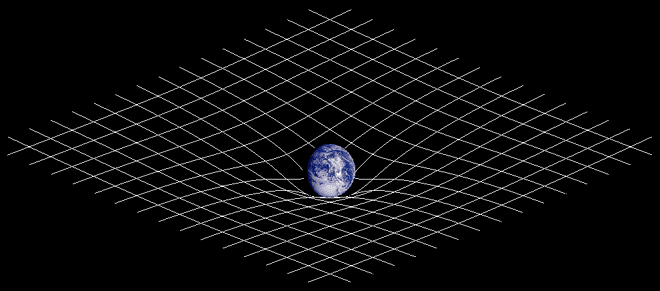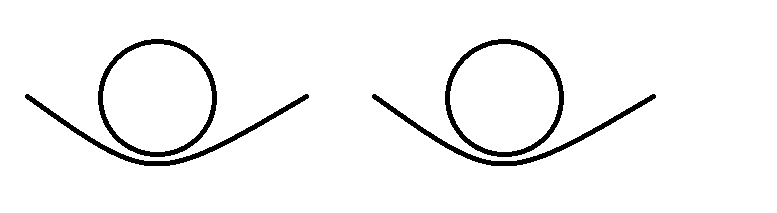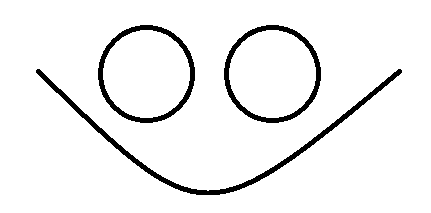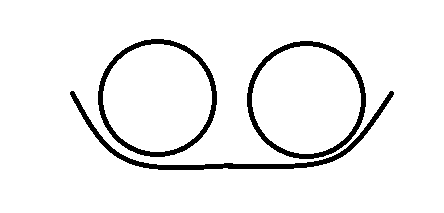# When gravity meets gravityAccording to what I understand about gravity in general relativity, is that it's a space time curvature (like the picture above).

I'm just a little curious. Let's say if there are two bodies with gravity with same mass, (assume two planets in 2D view)That (above) would be what the curvature look like when they're far enough.

But when they're about to collide into each other, what would happen to the curvature ?Would they form a constructive curve ? (Therefore increasing it's gravitational force isn't it ?)

OrWould they still collide but maintain both it's curvature ?

Thanks in advance.

*I wouldn't say I know much about general relativity

## Answers and Replies

Orodruin
Staff Emeritus
Science Advisor
Homework Helper
Gold Member
According to what I understand about gravity in general relativity, is that it's a space time curvature (like the picture above).

Yes, it is space-time curvature but no, it is not like the picture, which only shows spatial curvature.

Would they still collide but maintain both it's curvature ?

Einstein's equations are non-linear so yes, gravitation does not simply add up as a superposition. However, in most cases you will encounter, only the linear terms are relevant.

Yes, it is space-time curvature but no, it is not like the picture, which only shows spatial curvature.

Einstein's equations are non-linear so yes, gravitation does not simply add up as a superposition. However, in most cases you will encounter, only the linear terms are relevant.

Thank you so much. Just the answer I needed.